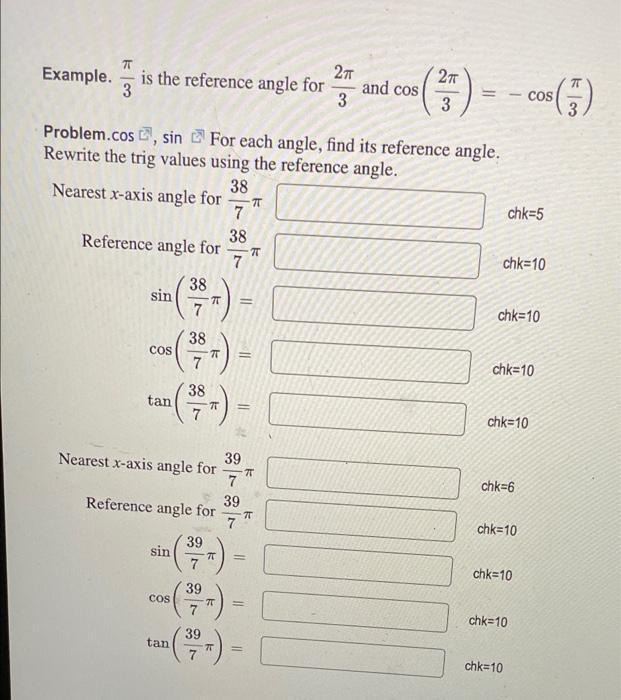Question:

# π/3 is the reference angle for 2π/3 and cos (2π/3) = -cos(π/3) For each angle, find its reference angle. Rewrite the trig values using the reference angle. (A) Nearest x-axis angle for 38/7π (B) Referπ/3 is the reference angle for 2π/3 and cos (2π/3) = -cos(π/3) For each angle, find its reference angle. Rewrite the trig values using the reference angle. (A) Nearest x-axis angle for 38/7π (B) Reference angle for 38/7π (C) sin (38/7π) = (D) cos (38/7π) = (E) tan (38/7π) = (F) Nearest x-axis angle for 39/7π (G) Reference angle for 39/7π (H) sin (39/7π) = (I) cos (39/7π) = (J) tan (39/7π) =